# Circular Singly Linked List | Insertion

We have discussed Singly and Circular Linked List in the following post:
Why Circular? In a singly linked list, for accessing any node of linked list, we start traversing from the first node. If we are at any node in the middle of the list, then it is not possible to access nodes that precede the given node. This problem can be solved by slightly altering the structure of singly linked list. In a singly linked list, next part (pointer to next node) is NULL, if we utilize this link to point to the first node then we can reach preceding nodes. Refer this for more advantages of circular linked lists.
The structure thus formed is circular singly linked list look like this: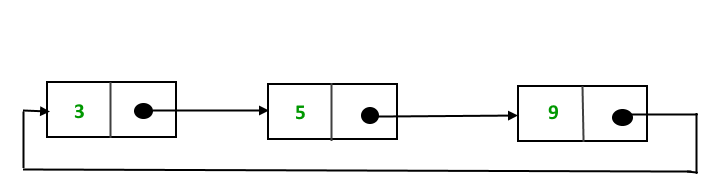In this post, the implementation and insertion of a node in a Circular Linked List using singly linked list are explained.

Implementation
To implement a circular singly linked list, we take an external pointer that points to the last node of the list. If we have a pointer last pointing to the last node, then last -> next will point to the first node.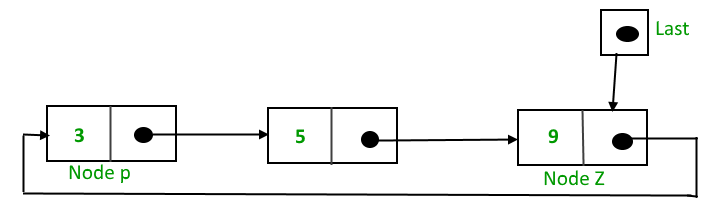The pointer last points to node Z and last -> next points to node P.

Why have we taken a pointer that points to the last node instead of first node ?
For insertion of node in the beginning we need traverse the whole list. Also, for insertion at the end, the whole list has to be traversed. If instead of start pointer we take a pointer to the last node then in both the cases there won’t be any need to traverse the whole list. So insertion in the begging or at the end takes constant time irrespective of the length of the list.

Insertion
A node can be added in three ways:

• Insertion in an empty list
• Insertion at the beginning of the list
• Insertion at the end of the list
• Insertion in between the nodes

Insertion in an empty List
Initially, when the list is empty, last pointer will be NULL.After inserting a node T,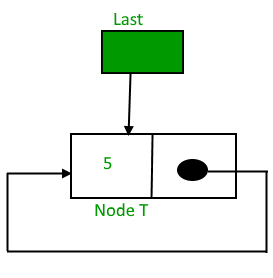After insertion, T is the last node so pointer last points to node T. And Node T is first and last node, so T is pointing to itself.
Function to insert node in an empty List,

## C++

 `struct` `Node *addToEmpty(``struct` `Node *last, ``int` `data)` `{` `    ``// This function is only for empty list` `    ``if` `(last != NULL)` `      ``return` `last;`   `    ``// Creating a node dynamically.` `    ``struct` `Node *last =` `          ``(``struct` `Node*)``malloc``(``sizeof``(``struct` `Node));`   `    ``// Assigning the data.` `    ``last -> data = data;`   `    ``// Note : list was empty. We link single node` `    ``// to itself.` `    ``last -> next = last;`   `    ``return` `last;` `}`

Insertion at the beginning of the list
To Insert a node at the beginning of the list, follow these step:
1. Create a node, say T.
2. Make T -> next = last -> next.
3. last -> next = T.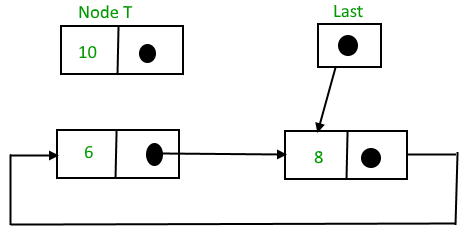After insertion,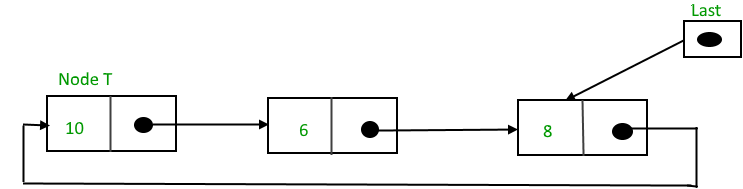Function to insert node in the beginning of the List,

## C++

 `struct` `Node *addBegin(``struct` `Node *last, ``int` `data)` `{` `  ``if` `(last == NULL)` `     ``return` `addToEmpty(last, data);`   `  ``// Creating a node dynamically.` `  ``struct` `Node *temp` `        ``= (``struct` `Node *)``malloc``(``sizeof``(``struct` `Node));` `  `  `  ``// Assigning the data.` `  ``temp -> data = data;`   `  ``// Adjusting the links.` `  ``temp -> next = last -> next;` `  ``last -> next = temp;` `  `  `  ``return` `last;` `}`

Insertion at the end of the list
To Insert a node at the end of the list, follow these step:
1. Create a node, say T.
2. Make T -> next = last -> next;
3. last -> next = T.
4. last = T.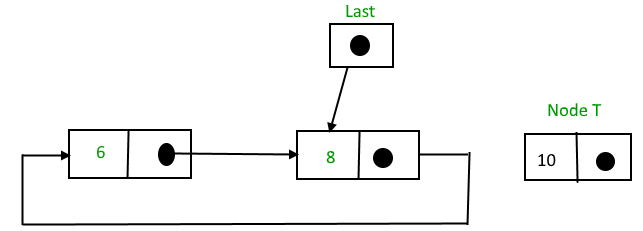After insertion,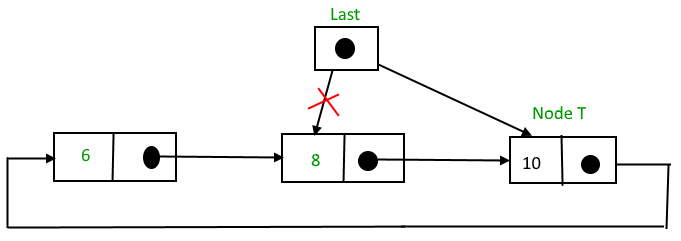Function to insert node in the end of the List,

## C++

 `struct` `Node *addEnd(``struct` `Node *last, ``int` `data)` `{` `  ``if` `(last == NULL)` `     ``return` `addToEmpty(last, data);`   `  ``// Creating a node dynamically.` `  ``struct` `Node *temp = ` `        ``(``struct` `Node *)``malloc``(``sizeof``(``struct` `Node));` `  `  `  ``// Assigning the data.` `  ``temp -> data = data;`   `  ``// Adjusting the links.` `  ``temp -> next = last -> next;` `  ``last -> next = temp;` `  ``last = temp;` `  `  `  ``return` `last;` `}`

Insertion in between the nodes
To Insert a node at the end of the list, follow these step:
1. Create a node, say T.
2. Search the node after which T need to be insert, say that node be P.
3. Make T -> next = P -> next;
4. P -> next = T.
Suppose 12 need to be insert after node having value 10,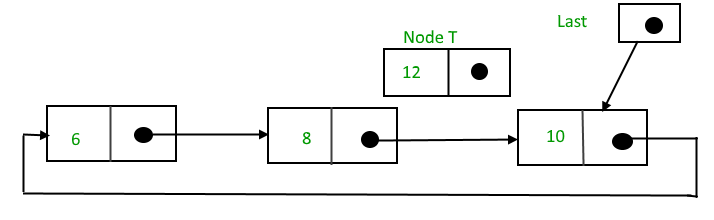After searching and insertion,Function to insert node in the end of the List,

## C++

 `struct` `Node *addAfter(``struct` `Node *last, ``int` `data, ``int` `item)` `{` `    ``if` `(last == NULL)` `       ``return` `NULL;`   `    ``struct` `Node *temp, *p;` `    ``p = last -> next;`   `    ``// Searching the item.` `    ``do` `    ``{` `        ``if` `(p ->data == item)` `        ``{` `            ``// Creating a node dynamically.` `            ``temp = (``struct` `Node *)``malloc``(``sizeof``(``struct` `Node));`   `            ``// Assigning the data.` `            ``temp -> data = data;`   `            ``// Adjusting the links.` `            ``temp -> next = p -> next;`   `            ``// Adding newly allocated node after p.` `            ``p -> next = temp;`   `            ``// Checking for the last node.` `            ``if` `(p == last)` `                ``last = temp;`   `            ``return` `last;` `        ``}` `        ``p = p -> next;` `    ``} ``while` `(p != last -> next);`   `    ``cout << item << ``" not present in the list."` `<< endl;` `    ``return` `last;` `}`

Following is a complete program that uses all of the above methods to create a circular singly linked list.

## C++

 `#include` `using` `namespace` `std;`   `struct` `Node` `{` `    ``int` `data;` `    ``struct` `Node *next;` `};`   `struct` `Node *addToEmpty(``struct` `Node *last, ``int` `data)` `{` `    ``// This function is only for empty list` `    ``if` `(last != NULL)` `      ``return` `last;`   `    ``// Creating a node dynamically.` `    ``struct` `Node *temp = ` `           ``(``struct` `Node*)``malloc``(``sizeof``(``struct` `Node));`   `    ``// Assigning the data.` `    ``temp -> data = data;` `    ``last = temp;`   `    ``// Creating the link.` `    ``last -> next = last;`   `    ``return` `last;` `}`   `struct` `Node *addBegin(``struct` `Node *last, ``int` `data)` `{` `    ``if` `(last == NULL)` `        ``return` `addToEmpty(last, data);`   `    ``struct` `Node *temp = ` `            ``(``struct` `Node *)``malloc``(``sizeof``(``struct` `Node));`   `    ``temp -> data = data;` `    ``temp -> next = last -> next;` `    ``last -> next = temp;`   `    ``return` `last;` `}`   `struct` `Node *addEnd(``struct` `Node *last, ``int` `data)` `{` `    ``if` `(last == NULL)` `        ``return` `addToEmpty(last, data);` `    `  `    ``struct` `Node *temp = ` `        ``(``struct` `Node *)``malloc``(``sizeof``(``struct` `Node));`   `    ``temp -> data = data;` `    ``temp -> next = last -> next;` `    ``last -> next = temp;` `    ``last = temp;`   `    ``return` `last;` `}`   `struct` `Node *addAfter(``struct` `Node *last, ``int` `data, ``int` `item)` `{` `    ``if` `(last == NULL)` `        ``return` `NULL;`   `    ``struct` `Node *temp, *p;` `    ``p = last -> next;` `    ``do` `    ``{` `        ``if` `(p ->data == item)` `        ``{` `            ``temp = (``struct` `Node *)``malloc``(``sizeof``(``struct` `Node));` `            ``temp -> data = data;` `            ``temp -> next = p -> next;` `            ``p -> next = temp;`   `            ``if` `(p == last)` `                ``last = temp;` `            ``return` `last;` `        ``}` `        ``p = p -> next;` `    ``}  ``while``(p != last -> next);`   `    ``cout << item << ``" not present in the list."` `<< endl;` `    ``return` `last;`   `}`   `void` `traverse(``struct` `Node *last)` `{` `    ``struct` `Node *p;`   `    ``// If list is empty, return.` `    ``if` `(last == NULL)` `    ``{` `        ``cout << ``"List is empty."` `<< endl;` `        ``return``;` `    ``}`   `    ``// Pointing to first Node of the list.` `    ``p = last -> next;`   `    ``// Traversing the list.` `    ``do` `    ``{` `        ``cout << p -> data << ``" "``;` `        ``p = p -> next;`   `    ``}` `    ``while``(p != last->next);`   `}`   `// Driven Program` `int` `main()` `{` `    ``struct` `Node *last = NULL;`   `    ``last = addToEmpty(last, 6);` `    ``last = addBegin(last, 4);` `    ``last = addBegin(last, 2);` `    ``last = addEnd(last, 8);` `    ``last = addEnd(last, 12);` `    ``last = addAfter(last, 10, 8);`   `    ``traverse(last);`   `    ``return` `0;` `}`

## Java

 `class` `GFG ` `{`   `static` `class` `Node` `{` `    ``int` `data;` `    ``Node next;` `};`   `static` `Node addToEmpty(Node last, ``int` `data)` `{` `    ``// This function is only for empty list` `    ``if` `(last != ``null``)` `    ``return` `last;`   `    ``// Creating a node dynamically.` `    ``Node temp = ``new` `Node();`   `    ``// Assigning the data.` `    ``temp.data = data;` `    ``last = temp;`   `    ``// Creating the link.` `    ``last.next = last;`   `    ``return` `last;` `}`   `static` `Node addBegin(Node last, ``int` `data)` `{` `    ``if` `(last == ``null``)` `        ``return` `addToEmpty(last, data);`   `    ``Node temp = ``new` `Node();`   `    ``temp.data = data;` `    ``temp.next = last.next;` `    ``last.next = temp;`   `    ``return` `last;` `}`   `static` `Node addEnd(Node last, ``int` `data)` `{` `    ``if` `(last == ``null``)` `        ``return` `addToEmpty(last, data);` `    `  `    ``Node temp = ``new` `Node();`   `    ``temp.data = data;` `    ``temp.next = last.next;` `    ``last.next = temp;` `    ``last = temp;`   `    ``return` `last;` `}`   `static` `Node addAfter(Node last, ``int` `data, ``int` `item)` `{` `    ``if` `(last == ``null``)` `        ``return` `null``;`   `    ``Node temp, p;` `    ``p = last.next;` `    ``do` `    ``{` `        ``if` `(p.data == item)` `        ``{` `            ``temp = ``new` `Node();` `            ``temp.data = data;` `            ``temp.next = p.next;` `            ``p.next = temp;`   `            ``if` `(p == last)` `                ``last = temp;` `            ``return` `last;` `        ``}` `        ``p = p.next;` `    ``} ``while``(p != last.next);`   `    ``System.out.println(item + ``" not present in the list."``);` `    ``return` `last;`   `}`   `static` `void` `traverse(Node last)` `{` `    ``Node p;`   `    ``// If list is empty, return.` `    ``if` `(last == ``null``)` `    ``{` `        ``System.out.println(``"List is empty."``);` `        ``return``;` `    ``}`   `    ``// Pointing to first Node of the list.` `    ``p = last.next;`   `    ``// Traversing the list.` `    ``do` `    ``{` `        ``System.out.print(p.data + ``" "``);` `        ``p = p.next;`   `    ``}` `    ``while``(p != last.next);`   `}`   `// Driven code` `public` `static` `void` `main(String[] args)` `{` `    ``Node last = ``null``;`   `    ``last = addToEmpty(last, ``6``);` `    ``last = addBegin(last, ``4``);` `    ``last = addBegin(last, ``2``);` `    ``last = addEnd(last, ``8``);` `    ``last = addEnd(last, ``12``);` `    ``last = addAfter(last, ``10``, ``8``);`   `    ``traverse(last);` `}` `}`   `/* This code contributed by PrinciRaj1992 */`

## Python3

 `class` `Node:` `    ``def` `__init__(``self``, data):` `        ``self``.data ``=` `data` `        ``self``.``next` `=` `None`   `class` `CircularLinkedList:` `    ``def` `__init__(``self``):` `        ``self``.last ``=` `None`   `    ``# This function is only for empty list` `    ``def` `addToEmpty(``self``, data):`   `        ``if` `(``self``.last !``=` `None``):` `            ``return` `self``.last`   `        ``# Creating the newnode temp` `        ``temp ``=` `Node(data)` `        ``self``.last ``=` `temp`   `        ``# Creating the link` `        ``self``.last.``next` `=` `self``.last` `        ``return` `self``.last`   `    ``def` `addBegin(``self``, data):`   `        ``if` `(``self``.last ``=``=` `None``):` `            ``return` `self``.addToEmpty(data)`   `        ``temp ``=` `Node(data)` `        ``temp.``next` `=` `self``.last.``next` `        ``self``.last.``next` `=` `temp`   `        ``return` `self``.last`   `    ``def` `addEnd(``self``, data):`   `        ``if` `(``self``.last ``=``=` `None``):` `            ``return` `self``.addToEmpty(data)`   `        ``temp ``=` `Node(data)` `        ``temp.``next` `=` `self``.last.``next` `        ``self``.last.``next` `=` `temp` `        ``self``.last ``=` `temp`   `        ``return` `self``.last`   `    ``def` `addAfter(``self``, data, item):`   `        ``if` `(``self``.last ``=``=` `None``):` `            ``return` `None`   `        ``temp ``=` `Node(data)` `        ``p ``=` `self``.last.``next` `        ``while` `p:` `            ``if` `(p.data ``=``=` `item):` `                ``temp.``next` `=` `p.``next` `                ``p.``next` `=` `temp`   `                ``if` `(p ``=``=` `self``.last):` `                    ``self``.last ``=` `temp` `                    ``return` `self``.last` `                ``else``:` `                    ``return` `self``.last` `            ``p ``=` `p.``next` `            ``if` `(p ``=``=` `self``.last.``next``):` `                ``print``(item, ``"not present in the list"``)` `                ``break`   `    ``def` `traverse(``self``):` `        ``if` `(``self``.last ``=``=` `None``):` `            ``print``(``"List is empty"``)` `            ``return`   `        ``temp ``=` `self``.last.``next` `        ``while` `temp:` `            ``print``(temp.data, end ``=` `" "``)` `            ``temp ``=` `temp.``next` `            ``if` `temp ``=``=` `self``.last.``next``:` `                ``break`   `# Driver Code` `if` `__name__ ``=``=` `'__main__'``:`   `    ``llist ``=` `CircularLinkedList()`   `    ``last ``=` `llist.addToEmpty(``6``)` `    ``last ``=` `llist.addBegin(``4``)` `    ``last ``=` `llist.addBegin(``2``)` `    ``last ``=` `llist.addEnd(``8``)` `    ``last ``=` `llist.addEnd(``12``)` `    ``last ``=` `llist.addAfter(``10``,``8``)`   `    ``llist.traverse()`   `# This code is contributed by` `# Aditya Singh`

## C#

 `using` `System;` `    `  `public` `class` `GFG ` `{` ` `  `public` `class` `Node` `{` `    ``public` `int` `data;` `    ``public` `Node next;` `};` ` `  `static` `Node addToEmpty(Node last, ``int` `data)` `{` `    ``// This function is only for empty list` `    ``if` `(last != ``null``)` `    ``return` `last;` ` `  `    ``// Creating a node dynamically.` `    ``Node temp = ``new` `Node();` ` `  `    ``// Assigning the data.` `    ``temp.data = data;` `    ``last = temp;` ` `  `    ``// Creating the link.` `    ``last.next = last;` ` `  `    ``return` `last;` `}` ` `  `static` `Node addBegin(Node last, ``int` `data)` `{` `    ``if` `(last == ``null``)` `        ``return` `addToEmpty(last, data);` ` `  `    ``Node temp = ``new` `Node();` ` `  `    ``temp.data = data;` `    ``temp.next = last.next;` `    ``last.next = temp;` ` `  `    ``return` `last;` `}` ` `  `static` `Node addEnd(Node last, ``int` `data)` `{` `    ``if` `(last == ``null``)` `        ``return` `addToEmpty(last, data);` `     `  `    ``Node temp = ``new` `Node();` ` `  `    ``temp.data = data;` `    ``temp.next = last.next;` `    ``last.next = temp;` `    ``last = temp;` ` `  `    ``return` `last;` `}` ` `  `static` `Node addAfter(Node last, ``int` `data, ``int` `item)` `{` `    ``if` `(last == ``null``)` `        ``return` `null``;` ` `  `    ``Node temp, p;` `    ``p = last.next;` `    ``do` `    ``{` `        ``if` `(p.data == item)` `        ``{` `            ``temp = ``new` `Node();` `            ``temp.data = data;` `            ``temp.next = p.next;` `            ``p.next = temp;` ` `  `            ``if` `(p == last)` `                ``last = temp;` `            ``return` `last;` `        ``}` `        ``p = p.next;` `    ``} ``while``(p != last.next);` ` `  `    ``Console.WriteLine(item + ``" not present in the list."``);` `    ``return` `last;` ` `  `}` ` `  `static` `void` `traverse(Node last)` `{` `    ``Node p;` ` `  `    ``// If list is empty, return.` `    ``if` `(last == ``null``)` `    ``{` `        ``Console.WriteLine(``"List is empty."``);` `        ``return``;` `    ``}` ` `  `    ``// Pointing to first Node of the list.` `    ``p = last.next;` ` `  `    ``// Traversing the list.` `    ``do` `    ``{` `        ``Console.Write(p.data + ``" "``);` `        ``p = p.next;` ` `  `    ``}` `    ``while``(p != last.next);` ` `  `}` ` `  `// Driven code` `public` `static` `void` `Main(String[] args)` `{` `    ``Node last = ``null``;` ` `  `    ``last = addToEmpty(last, 6);` `    ``last = addBegin(last, 4);` `    ``last = addBegin(last, 2);` `    ``last = addEnd(last, 8);` `    ``last = addEnd(last, 12);` `    ``last = addAfter(last, 10, 8);` ` `  `    ``traverse(last);` `}` `}` `// This code contributed by Rajput-Ji`

Output:

```2 4 6 8 10 12
```

This article is contributed by Anuj Chauhan. If you like GeeksforGeeks and would like to contribute, you can also write an article using contribute.geeksforgeeks.org or mail your article to contribute@geeksforgeeks.org. See your article appearing on the GeeksforGeeks main page and help other Geeks.